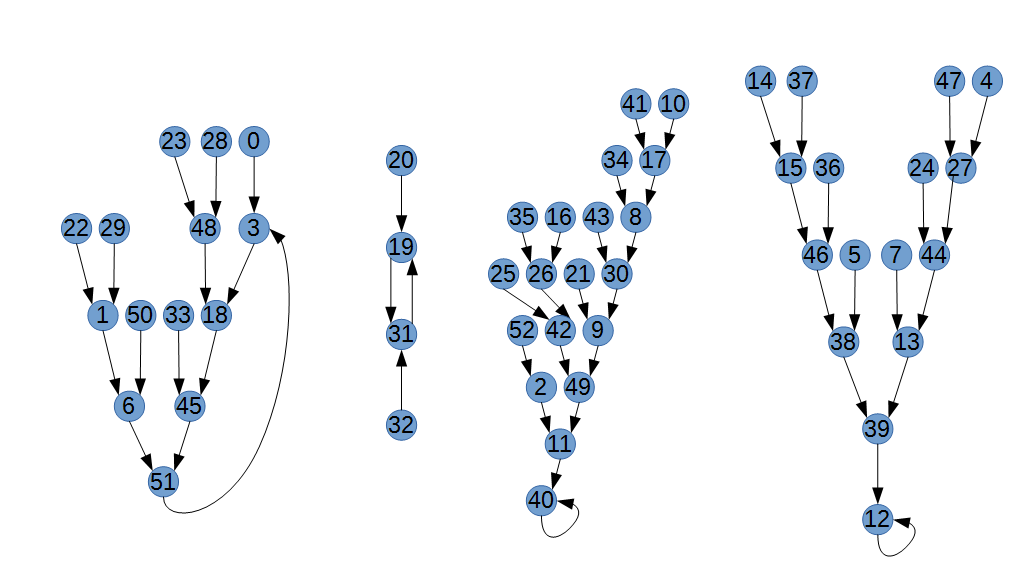# Symmetry in quadratic equations mod n

Inspired by this problem

The problem is about a quadratic function modulo 53, and it asks for which function values you can compose this function multiple times to get a result of 18.

What's interesting, is that the solutions come in pairs whose sum is always 51, and actually $\forall a,b \in (\mathbb{Z}/53\mathbb{Z}) (a+b=51 \Rightarrow f(a)=f(b))$ with the ring of integers modulo 53 $\mathbb{Z}/53\mathbb{Z}$.

Is this a consequence of mod 53 or always true for quadratic equations?

This is the graph that shows for each value what the function gives and where you would continue and it is really symmetric and I think beautiful (except for a few links that break the patterns)Proof

So this is a proof for this particular polynomial

$(51-n)^2+2(51-n)+3$

$= n^2-104n+2706$

$\equiv (n^2 \pmod {53}) + (-104n \pmod {53}) + (2706 \pmod {53})$

$\equiv n^2+2n+3 \pmod {53}$

and for some prime $p$

$((p-2)-n)^2+2((p-2)-n)+3$

$= n^2-2pn+2n+p^2-2p+3$

$\equiv (n^2 \pmod p) + (-2pn+2n \pmod p) + (p^2-2p+3 \pmod p) \equiv (n^2 \pmod p) + (2n \pmod p) + (3 \pmod p)$

$\equiv n^2+2n+3 \pmod p$

In general

For a general quadratic polynomial $an^2+bn+c \pmod p$ with $a,b,c \in \mathbb{N}, p \in \mathbb{P}, a \neq 0$

$a((p-2)-n)^2+b((p-2)-n)+c$

$= ((a)n^2+(4a-2ap-b)n+(ap^2+bp-4ap+c-2b+4a))$

$\equiv (an^2 \pmod p) + (4an-2apn-bn \pmod p) + (ap^2-4ap+bp+4a-2b+c \pmod p)$

$\equiv (an^2 \pmod p) + (4an-bn \pmod p) + (4a-2b+c \pmod p)$

$\equiv ((an^2) + (4an-bn) + (4a-2b+c)) \pmod p$

For this to be congruent to the original polynomial, we require

$a \equiv a \pmod p$

$4a-b \equiv b \pmod p$

$4a-2b+c \equiv c \pmod p$

The first condition is always true, the other two are equivalent and can be rewritten as

$2a \equiv b \pmod p$

So $c$ actually has no influence and we have only one condition on the coefficients (and $p$ must be prime).

Degree 4

Let's try to do the same thing with a polynomial of degree 4 (because 3 doesn't work well), so $an^4+bn^3+cn^2+dn+e$ with $a,b,c,d,e \in \mathbb{N}, p \in \mathbb{P}, a \neq 0$

$a(p-2-n)^4+b(p-2-n)^3+c(p-2-n)^2+d(p-2-n)+e$

$= (\text{stuff}) \cdot p + an^4 + 8an^3-bn^3 + 24an^2-6bn^2+cn^2 + 32an-12bn+4cn-dn + 16a-8b+4c-2d+e$

$\equiv ((a)n^4 + (8a-b)n^3 + (24a-6b+c)n^2 + (32a-12b+4c-d)n + (16a-8b+4c-2d+e)) \pmod p$

By equating coefficients, we get

$a \equiv a \pmod p$

$8a-b \equiv b \pmod p$

$24a-6b+c \equiv c \pmod p$

$32a-12b+4c-d \equiv d \pmod p$

$16a-8b+4c-2d+e \equiv \pmod p$

Again, the first congruence is trivial and the second and third are equivalent. By pluging the second into the fourth and fifth, we find that they are also equivalent. So, we end up with two congruences

$4a-b \equiv 0 \pmod p$

$-2b+2c-d \equiv 0 \pmod p$

If we want we can pick some numbers, maybe $a=1$ to have a normalized polynomial, then $b$ has to be $4$. For $c$ and $d$, we can pick $5$ and $2$ and since $e$ is without any restriction, let's make it nice and take $e=3$. This gives the polynomial

$f(n) = n^4+4n^3+5n^2+2n+3$

and if we try we see that it really follows our rule

$\forall a,b \in (\mathbb{Z}/53\mathbb{Z}) (a+b=51 \Rightarrow f(a)=f(b))$Note by Henry U
2 years, 9 months ago

This discussion board is a place to discuss our Daily Challenges and the math and science related to those challenges. Explanations are more than just a solution — they should explain the steps and thinking strategies that you used to obtain the solution. Comments should further the discussion of math and science.

When posting on Brilliant:

• Use the emojis to react to an explanation, whether you're congratulating a job well done , or just really confused .
• Ask specific questions about the challenge or the steps in somebody's explanation. Well-posed questions can add a lot to the discussion, but posting "I don't understand!" doesn't help anyone.
• Try to contribute something new to the discussion, whether it is an extension, generalization or other idea related to the challenge.

MarkdownAppears as
*italics* or _italics_ italics
**bold** or __bold__ bold
- bulleted- list
• bulleted
• list
1. numbered2. list
1. numbered
2. list
Note: you must add a full line of space before and after lists for them to show up correctly
paragraph 1paragraph 2

paragraph 1

paragraph 2

[example link](https://brilliant.org)example link
> This is a quote
This is a quote
    # I indented these lines
# 4 spaces, and now they show
# up as a code block.

print "hello world"
# I indented these lines
# 4 spaces, and now they show
# up as a code block.

print "hello world"
MathAppears as
Remember to wrap math in $$ ... $$ or $ ... $ to ensure proper formatting.
2 \times 3 $2 \times 3$
2^{34} $2^{34}$
a_{i-1} $a_{i-1}$
\frac{2}{3} $\frac{2}{3}$
\sqrt{2} $\sqrt{2}$
\sum_{i=1}^3 $\sum_{i=1}^3$
\sin \theta $\sin \theta$
\boxed{123} $\boxed{123}$